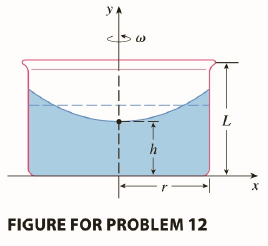Chapter 5.P, Problem 12P

Chapter
Section
Textbook Problem

# A cylindrical container of radius r and height L is partially filled with a liquid whose volume is V. If the container is rotated about its axis of symmetry with constant angular speed ω , then the container will induce a rotational motion in the liquid around the same axis. Eventually, the liquid will be rotating at the same angular speed as the container. The surface of the liquid will be convex, as indicated in the figure, because the centrifugal force on the liquid particles increases with the distance from the axis of the container. It can be shown that the surface of the liquid is a paraboloid of revolution generated by rotating the parabola y = h + ω 2 x 2 2 g about the y -axis, where g is the acceleration due to gravity.(a) Determine h as a function of ω .(b) At what angular speed will the surface of the liquid touch the bottom? At what speed will it spill over the top?(c) Suppose the radius of the container is 2 ft, the height is 7 ft, and the container and liquid are rotating at the same constant angular speed. The surface of the liquid is 5 ft below the top of the tank at the central axis and 4 ft below the top of the tank 1 ft out from the central axis.(i) Determine the angular speed of the container and the volume of the fluid.(ii) How far below the top of the tank is the liquid at the wall of the container?To determine

(a)

h as a function ω

Explanation

1) Concept:

The Cylindrical Shell method:

The volume of a solid obtained by rotating about the y axis the region under the curve y=f(x) from a to b is

V=ab2πxf(x) dx

2) Given:

y=h+ω2x22g

3) Calculation:

It is difficult to determine the inner and outer radius to use the washer method. Therefore, use the cylindrical method, and rotation about y axis.

By using cylindrical shell method, the shell has radius x, circumference 2πx, and height y. So the volume is

V=ab2πxf(x) dx=0r2πxh+ω2x22g dx

=2π·0rhx+ω2x32g dx

=2πhx22+ω2x48g0r

=2πhr2

To determine

(b)

To find:

At what speed will the surface of the liquid touch the bottom and the top?

To determine

(c)

To find:

i) The angular speed of container and volume of the fluid

ii) Liquid at the wall of the container below the top of tank

### Still sussing out bartleby?

Check out a sample textbook solution.

See a sample solution

#### The Solution to Your Study Problems

Bartleby provides explanations to thousands of textbook problems written by our experts, many with advanced degrees!

Get Started

#### find the real roots of each equation by factoring. 129. x2 + x 12 = 0

Applied Calculus for the Managerial, Life, and Social Sciences: A Brief Approach

#### Evaluate the integral. 8. tsintcostdt

Single Variable Calculus: Early Transcendentals

#### Suppose . Then F(x)g(x) + C f′(g(x)) + C F(g′(x)) + C F(g(x)) + C

Study Guide for Stewart's Single Variable Calculus: Early Transcendentals, 8th